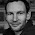## October 3, 2016

### A Quick View on Compositionality in Graph Pattern Matching

Graph pattern matching is the task of finding all matches (occurrences) of a pattern graph P in a host graph G. Formally, the goal is to compute the set of matches given by {m: P → G | m is a graph homomorphism}. In categorical terms, this is the set Hom(P,G). If we fix the host graph G, we get the functor Hom(_,G): Graph → Set. For any pattern P, Hom(_,G) sends P to the set of matches of P in G. The functor Hom(_,G) is contravariant. Thus, embedding a pattern P in Q means the match set of Q is included in P (modulo a translation of P into Q). In other words, every match of Q in G can be translated into a match of P in G

One view of compositionality for graph pattern matching is to decompose a pattern P into a span P2 ← P1 → P3 such that P is the pushout over this span. What does this mean for the corresponding match sets? Well, that's easy to answer now since we know we are dealing with Hom(_,G). If we look at the properties of this functor, we see that it sends colimits to limits. This means also that it maps a pushout of pattern graphs to a pullback of their corresponding match sets. Therefore, we can decompose the pattern matching task by finding the respective match sets of P1, P2 and P3 individually and composing them to the final match set Hom(P2,G) ×Hom(P1,G) Hom(P3,G). With some basic notions from category theory we achieved a quick compositionality result for graph pattern matching, neat.

What is this useful for? It can be used as a basis for operationalization and optimization of graph pattern matching. This has applications in graph databases and model transformations. For scalability of graph pattern matching w.r.t. the size of the host graph, the covariant functor Hom(P,_) is also very interesting.

The observation that a structural model (in this case pattern graphs) is related to its corresponding semantical model (in this case match sets) by a contravariant functor is not at all uncommon. Structure and semantics of behavioral models like Petri nets or Reo connectors are related in a similar way, as they form pairs of adjoint functors. This is not surprising because, as Saunders Mac Lane put it: ‘adjoint functors arise everywhere’.

#### 3 comments:

1.Hi Christian! Nice stuff, I like the clever proof for the compositionality result.

I wonder, though, if we can actually expect practical performance benefits from decomposing P into multiple sub-patterns. The reason for my doubt is that current graph pattern matching engines are less efficient at matching and combining multiple isolated patterns than at finding one large pattern: the latter problem seems inherently easier, since being able to navigate along the adjacent edges of partial solutions can help us to reduce the search space. I think you described this idea in your AMT2013 paper, in the explanation for the "LHS not connected" smell. But maybe you have some situations in mind where decomposing would indeed make things faster?

2.Graph pattern matching engines usually use some kind of search plan. The decomposition of a pattern into a multiple subpatterns together with the order how these subpatterns are being matched is essentially the search plan. For example, consider a pattern of three nodes and two edges: A->B->C. Two possible decompositions of this pattern are (((A)->B)->C) and (A->(B->(C))). In the first decomposition we first match A, then B, then C. In the second, we do it the other way around. Both decompositions can be described as a diagram in GRAPH plus an order on how they are glued together to form the complete pattern. If the engine does not support traversing edges in backward direction, the first decomposition is much more efficient of course. However, if you have an attribute constraint on C and you can traverse in backward direction, the second decomposition can be more efficient. It is the task of the optimizer to find out which plan is the best.

3.Thanks for clarifying about the search plans. I initially understood the compositionality result as a formal underpinning for one particular search plan - in your example, the span (A<-B) <- B -> (B->C), which did not seem to be most efficient. But I now see that by considering larger diagrams, with pushouts over pushouts, you can indeed represent arbitary search plans using this approach. Nice!